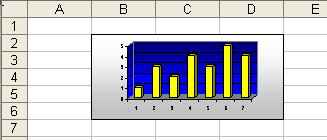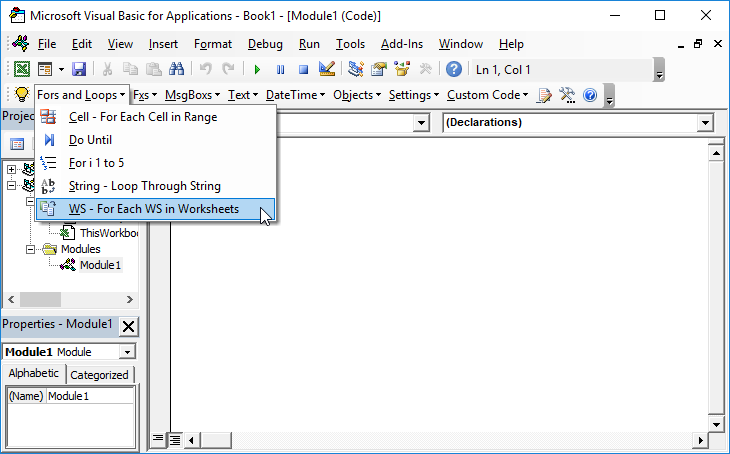# VBA – Resize an Object to a Range Size

## Resize Object to Range Size in VBA

You can size an object like Pictures, Autoshapes, and Charts to be the same size as a Range. To do this, set the objects .Left .Top .Width and .Height properties equal to the respective properties of a Range.

The following example sizes a Chart to the Range B2:D6The VBA code used to accomplish this:

``````Sub SizeChart2Range()

Dim MyChart As Chart

Dim MyRange As Range

Set MyChart = ActiveSheet.ChartObjects(1).Chart

Set MyRange = Sheet1.Range("B2:D6")

With MyChart.Parent

.Left = MyRange.Left

.Top = MyRange.Top

.Width = MyRange.Width

.Height = MyRange.Height

End With

End Sub``````